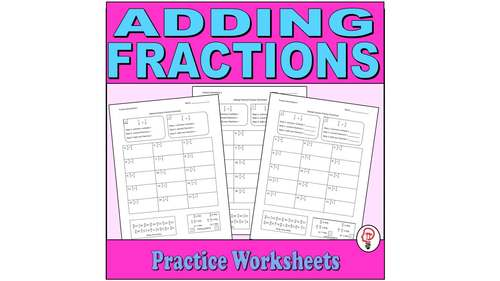# Adding Fractions - Practice WorksheetsSubject
Resource Type
File Type

Zip

(334 KB|3 pages)
Product Rating
Standards
• Product Description
• StandardsNEW

3 Practice worksheets on adding fractions.

Each worksheet has 2 guided practice problems with step by step aids to help students correctly solve the problems. 15 questions with answers provided at the bottom so students can check their work. The back of each has 18 problems for independent work and assessment.

Numerators are all less than 9, answers are never equal to or greater than 1. The focus is on converting fractions to common denominators, adding, and simplifying. No word problems - only skills development.

Add and subtract fractions with unlike denominators (including mixed numbers) by replacing given fractions with equivalent fractions in such a way as to produce an equivalent sum or difference of fractions with like denominators. For example, 2/3 + 5/4 = 8/12 + 15/12 = 23/12. (In general, 𝘢/𝘣 + 𝘤/𝘥 = (𝘢𝘥 + 𝘣𝘤)/𝘣𝘥.)
Total Pages
3 pages
Included
Teaching Duration
N/A
Report this Resource to TpT
Reported resources will be reviewed by our team. Report this resource to let us know if this resource violates TpT’s content guidelines.